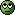# Understanding MAX in SQL with PHP

Hello!
I’m trying to pull off the largest index for a particular group (the \$user_id’s each have several \$course_id’s associated with them). I’m using the following PHP/SQL to get the info from my database:

``````function getlastCourseTaught(\$read,\$user_id) {
\$sql = "SELECT MAX(course_id)
FROM courses
WHERE user_id = \$user_id";
}
``````

The code works but I’m having problems getting at the actual ID. When I print_r my results, I get:

Array (  => Array ( [MAX(course_id)] => 1 ) )

So, two questions:

1. Why is SQL giving me back an array? Doesn’t the MAX just return one specific number?
2. How can I untangle the “array within the array” to get at the MAX(course_id) of “1”.

Thanks!
-Eric

PS IF this thread is more of a PHP question, please move it by all means.MySQL returns a result set: a number of rows, and each row contains a number of columns.
The fetchAll function you’re calling is apparently transforming the MySQL result set in a multidimensional array: an array that contains the rows, and each row-element is an array that contains the columns.

In this case, the result is an array of 1 row, that contains an array of 1 column.

To get the number, first of all I’d use an alias in the query:

``````SELECT MAX(course_id) [B][COLOR="Red"]AS maxid[/COLOR][/B]
``````

``````